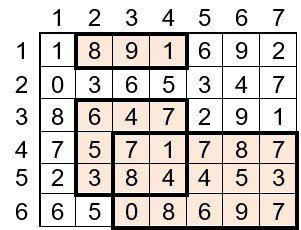Problems

# Demining

After the fighting, it became necessary to demine the area. It is known that the terrain is represented by a rectangular area measuring n by m, which may contain mines. The number of mines located in the corresponding terrain sector is represented by an integer xij (0xij109).

The military using a quadrocopter takes pictures of the area. Help them count the number of mines that are captured by the quadcopter if the quadcopter took k rectangular pictures of the area.

#### Input data

The first line contains two positive integers n and m (1n, m1000) - the number of rows and columns respectively.

The next n lines contain m integers xij (0xij109) - the number of mines in the corresponding sector.

The next line contains the number k (1k1000) - the number of shots taken from the quadcopter.

Next k lines contain the coordinates of rectangular images (four numbers each): y1, y2, x1, x2, where

y1 is the line number where the snapshot starts,

y2 is the line number where the snapshot ends,

x1 is the column number where the snapshot starts,

x2 is the column number where the snapshot ends.

#### Output

Print a single number - the total number of mines that will be included in the pictures. Mines present on several pictures are counted once.Time limit 1 second
Memory limit 128 MiB
Input example #1
6 7
1 8 9 1 6 9 2
0 3 6 5 3 4 7
8 6 4 7 2 9 1
7 5 7 1 7 8 7
2 3 8 4 4 5 3
6 5 0 8 6 9 7
3
1 1 2 4
3 5 2 4
4 6 3 7

Output example #1
127

Source ІІ stage of All-Ukrainian informatics olympiad in Zhitomyr region 17.12.2022